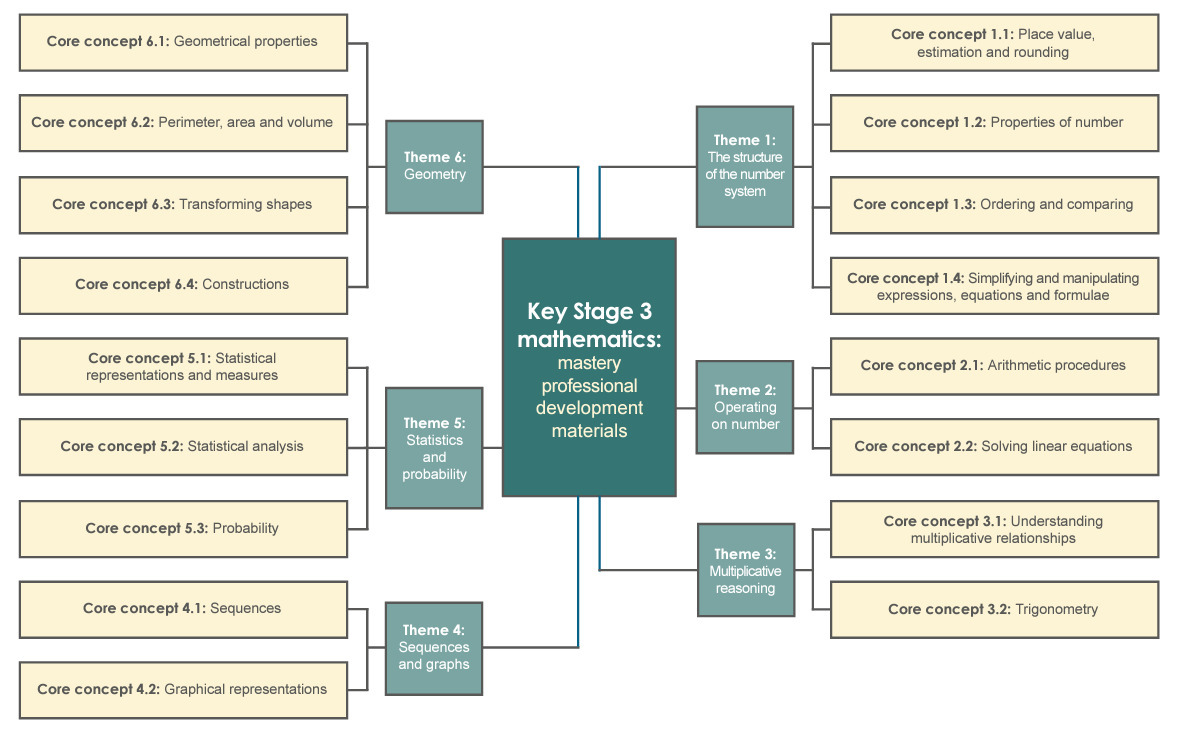# Department - Maths

Staff list

Mr A Haghazali - Associate Senior Leader Mathematics
Ms S Wells - Deputy Learning Leader (PP focus) Mathematics
Mr P Shand - Deputy Learning Leader Mathematics
Mrs P Bentham - Teacher of Mathematics / Head of Year 10
Mrs A Bhushan - Teacher of Mathematics
Mrs E Lipscombe-Holmes - Teacher of Mathematics
Mr P Okello - Teacher of Mathematics
Miss M Romero - Teacher of Mathematics
Mr A Morris - Teacher of Mathematics & Learning Leader Business Studies

Vision

The aim of the curriculum is to prepare all students with the mathematical knowledge for GCSE and also support students in their journey to become numerate, better problem solvers in mathematical contexts and in real life contexts. Have the ability to critique and analyse the events in the world and participate in democratic processes better informed. Experience and appreciate the beauty of mathematics in its own right as the language of nature and universe.

Intent

Content Covers 6 different areas of Mathematics: Number, Algebra, Ratio, proportion and rates of change, Geometry and measures, Probability and Statistics. Each topic in the content is met at a new level as the course progresses. The course is intended to prepare the students with the skills required for GCSE in Mathematics and skills needed later in life. Pupils continue to extend their understanding of various parts of the curriculum as they move from Year 7 to Year 9. Learning Activities Involved are under pinned by students participating in:

• Listening: to teacher when explanations are given, when mathematical methods are modelled and when peers are contributing.
• Thinking and Doing: Practicing and thinking about the processes and checking understanding.
• Discussing: Peer discussion and group discussion that supports learning. Students would be expected to demonstrate their understanding and mathematical reasoning. As part of the learning process students will be exposed to problem solving. Investigating different parts of mathematics.

Lessons to include demonstration and modelling of learning objectives, introduction of new mathematical vocabulary.

Impact

Understanding of mathematical concepts. Mastery of the concepts in problem solving. Application of mathematics. Teaching, Assessment, Planning, Modelling examples, Modelling mathematical thinking in solving problems. Students become more confident in Mathematics. Students to see mathematics as useful.